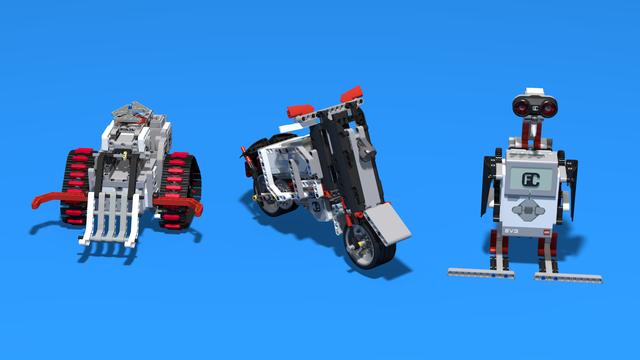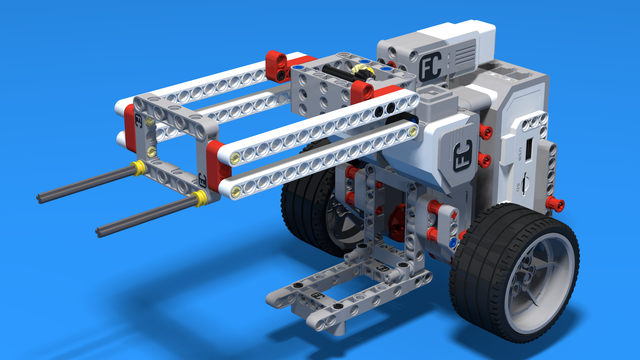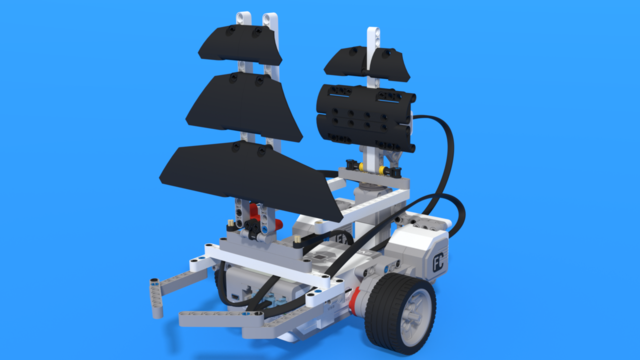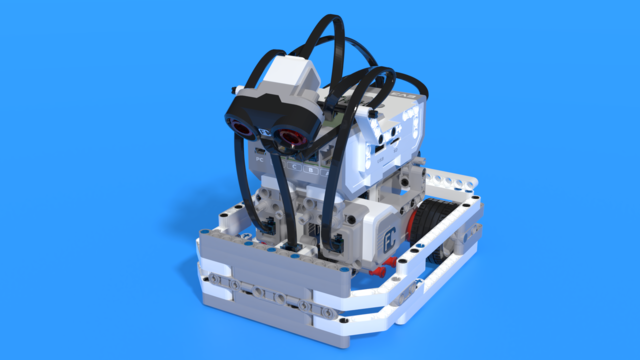# How to on Gears (part 1)

Gears could be a powerful tool for those who know how to use them appropriately. In this episode we introduce some of the basic terms and concepts behind using gears in your construction.

• #54
• 26 Sep 2014
• 5:17

Each mechanism you build has its unique needs, based on its aim. In some cases, you need more speed and in others - you need more torque or power. In some cases, you need a linear motion and in others - it should be circular. To achieve that you need to build a system of gears that will fit your needs. In this part of the gear tutorial we will cover and explain some of the basic terms behind the physics of gear systems.

### Speed

First of all, let's take a look at the term "speed" and explain the difference between linear and rotational speed:

• linear speed is the distance an object travels for a certain amount of time. It is measured in kph, mph, m/s etc. Knowing the speed of an object enables us to calculate the distance it will travel. For example, if a car is traveling at a speed of 50 kph, then it will travel 100 km in two hours.
• rotational speed is the number of rotations an object makes for a certain amount of time. Usually, it is measured in rotations per minute or RMP. For instance, the Large LEGO EV3 Motor has a rotational speed of 150RPM when set to 100% power. Hence, if we program it to rotate for 120 seconds or 2 minutes, it will rotate 300 times.

### Torque

After we have learned what speed is, we will move on to the next key characteristic - torque. Torque is a rotational force. Simply put, it is the force you have to apply to stop an object rotating. The larger the torque of a motor is, the greater the force you will need to stop it.

For example, if we want a robot to climb on an inclined plane or surface, or if we want it to pull a large load, we will need more torque. On the other hand, if we want our robot to compete, we will need more speed.

### Mechanical Power

The last concept we will cover is mechanical power. For our purposes, we can consider the following:

Mechanical Power  = Rotational Speed X Torque

When we set the power of the motors from the Move Blocks, we actually set the mechanical power. So, when we increase the value, we not only increase the speed of the motor but also we increase its torque.

### Gear Wheels

Now, we are ready to put into practice what we have learned so far. Gear is the group of gear wheels that engage and hence drive each other. The gear wheel that is directly driven by the motor is called a driver gear, while the one that is attached to the wheels, or the output gear, is called a follower gear.

Each gear wheel has multiple teeth on its circumference. When we put two gear wheels next to each other, the teeth engage. As a result, when we rotate one of the two gear wheels, the other one also rotates. Interestingly, every time a tooth from the first gear passes through the engage point, a tooth from the second gear will also pass.

To see this in practice, construct the gear following the instructions in the video.

### English

Each mechanism you build has its unique needs based on its aim. In some cases you need more speed, in others you need more torque or power. In some cases you need a linear motion and in others it should be rotational. To achieve this you need to build gear systems to modify the motion from the motors so that it will fit your needs. Stay with me for the next few minutes when I'll cover some of the basics of gear systems.

First of all, let's get familiar with some of the terms we'll be using from now on. Speed is a key characteristic of the gear systems. Usually, the term speed means distance an object travels for a certain amount of time. This actually is linear speed. The term rotational speed will be more important to us. This is the number of rotations an object does for a certain amount of time. Usually, it is measured in rotations per minute or, briefly, just RPM. Just an example: the EV3 large motor does about 150 RPM when using the rechargeable battery and is set at 100% power. We could easily check this with this simple program. First, we have motor A moving for 60 seconds at 100% power. Afterwards, we measure how many rotations it has made using the rotation sensor and display the result. Finally, we leave a ten-second pause so we could see the result. After running the program, you should get the result closer to 150. You could run this program several times in order to find the average speed of the motor. Just as you measure the force applied to an object to make it move in some direction, we'd like to measure the force applied to an object to make it rotate. The last is what the term torque actually means. The greater the torque is, the harder it'll be for the object to stop rotating. For instance, if you make a robot that has to climb up an incline, you'll need to have greater torque applied to its wheels. The next term we'll take a look at is mechanical power. For our purposes this will be the rotational speed multiplied by torque. The power you set in the settings of the move blocks is actually the mechanical power of the motor. For convenience, I will refer to it as just power. Now, let's take a look at a simple gear system. The red gear is directly attached to the motor via an axle. This is the input gear and I'll refer to it as driver gear. The blue one which is connected direcly to the tyre, or the one that outputs the motion from the gear system, will be called follower gear. Now let's see the gear system in motion. As you can see, the follower gear rotates much faster than the driver gear. You can count the teeth of the driver gear or just trust me that they are 40. The follower gear has only 8 teeth. So, for one rotation of the driver gear 40 teeth will go through the point of the engage and for each tooth of the driver gear that passes through that point a tooth of the follower gear should pass. But the follower gear has only 8 teeth, so for one rotation of the red gear which has 40 teeth it will make five rotations. This means we have increased the rotational speed five times. As I mentioned, the rotational speed multiplied by the torque gives the power. The power is constant so it does not change. So, the equation is still true, even if we have increased the rotational speed. This means we have decreased the torque five times. So, in this system, we inrease the speed and decrease the torque. In such cases we say that we are doing a gear up. In the opposite situation when the smaller gear, the one with the fewer teeth, is the driver gear and the bigger gear is the follower gear, we'll decrease the speed and increase the torque. In that case we are doing a gear down. Finally, let's put into practice what we have learnt so far. Here I have three five-minute bots. The first one has no gear systems. The second one is geared down and the last one is geared up. All three robots are set to move forward for 1 second at 100% power. First, let's run the geared-up one.

It reached this far but returned a bit because of the inertia and backlash - terms we'll be discussing in future tutorials.

Next, we have the geared-down robot. This robot has much more torque but traveled far less distance than the geared-up robot.

Finally, we have the robot without any gears. It traveled more distance than the geared-down robot but a third of the distance traveled by the geared-up robot. We'll stop here with the first part of the gear tutorial.

## Courses and lessons with this Tutorial

This Tutorial is used in the following courses and lessons• 135
• 280:11
• 156• 60
• 347:26
• 0• 6
• 0
• 0
• 3d_rotation 8• 26
• 98:39
• 47• 8
• 0
• 3
• 3d_rotation 1• 37
• 193:26
• 30• 2
• 0
• 4
• 3d_rotation 3• 37
• 6:37
• 111• 3
• 3
• 2
• 3d_rotation 2• 56
• 12:47
• 135• 4
• 3
• 2
• 3d_rotation 2• 49
• 58:06
• 32• 34
• 13:40
• 194